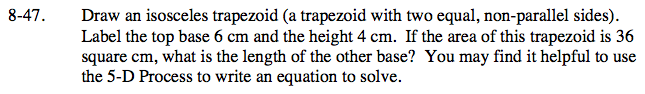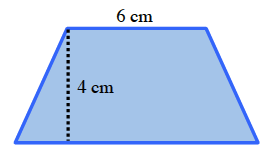### Home > CC2 > Chapter 8 > Lesson 8.2.2 > Problem8-47

8-47.

8‑47. Draw an isosceles trapezoid (a trapezoid with two equal, non-parallel sides). Label the top base 6 cm and the height 4 cm. If the area of this trapezoid is 36 square cm, what is the length of the other base? You may find it helpful to use the 5‑D Process to write an equation to solve. Homework Help ✎Note: Figure not necessarily drawn to scale.

Try different values to solve for the other base.

$\text{Area}=\frac{1}{2}\cdot(b_1+b_2)\cdot h$

12 cm. Be sure to follow the Order of Operations.